# Spectral Clustering Royi Itzhak Spectral Clustering Algorithms that

• Slides: 51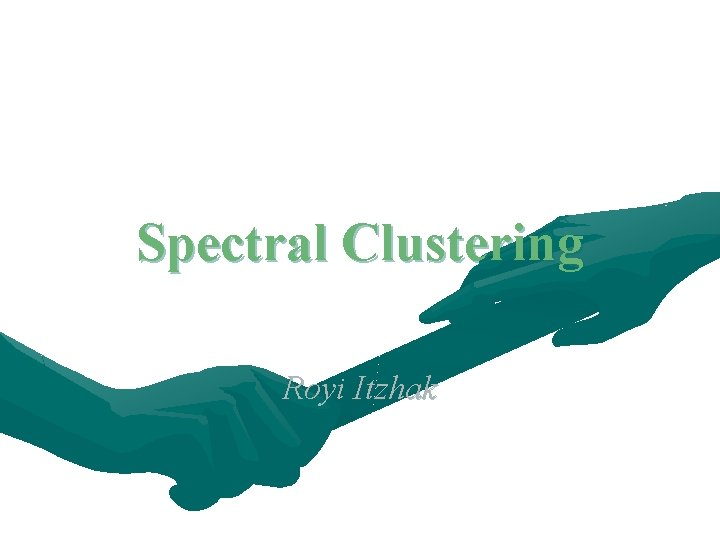Spectral Clustering Royi ItzhakSpectral Clustering • Algorithms that cluster points using eigenvectors of matrices derived from the data • Obtain data representation in the lowdimensional space that can be easily clustered • Variety of methods that use the eigenvectors differently • Difficult to understand….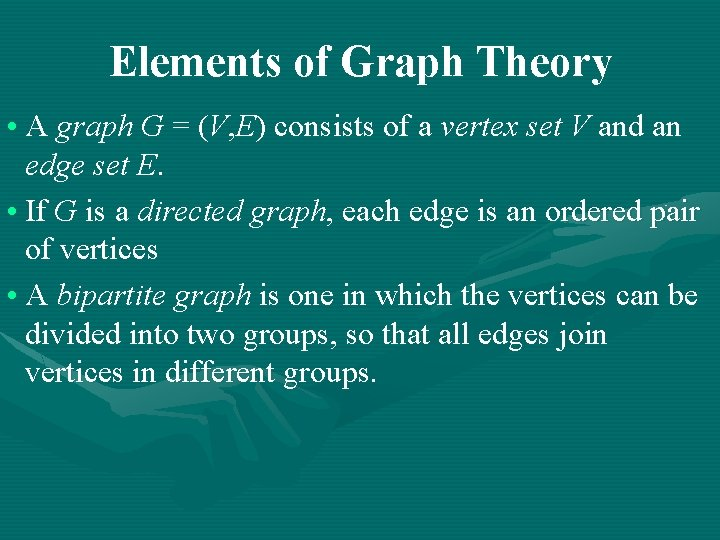Elements of Graph Theory • A graph G = (V, E) consists of a vertex set V and an edge set E. • If G is a directed graph, each edge is an ordered pair of vertices • A bipartite graph is one in which the vertices can be divided into two groups, so that all edges join vertices in different groups.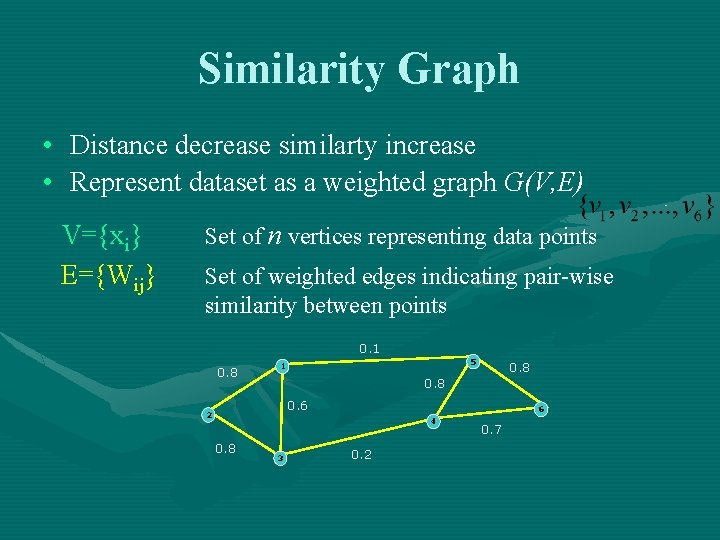Similarity Graph • Distance decrease similarty increase • Represent dataset as a weighted graph G(V, E) V={xi} E={Wij} Set of n vertices representing data points Set of weighted edges indicating pair-wise similarity between points 0. 1 0. 8 5 1 0. 8 0. 6 2 6 4 0. 8 3 0. 2 0. 7Similarity Graph • Wij represent similarity between vertex • If Wij=0 where isn’t similarity • Wii=0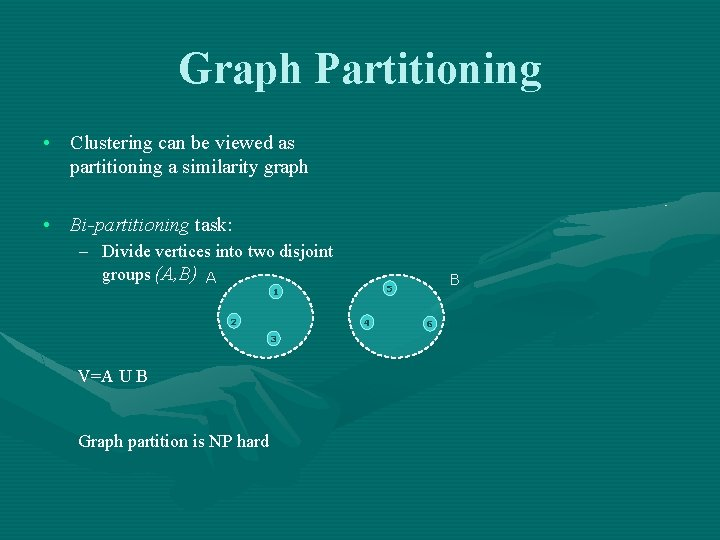Graph Partitioning • Clustering can be viewed as partitioning a similarity graph • Bi-partitioning task: – Divide vertices into two disjoint groups (A, B) A 2 4 3 V=A U B Graph partition is NP hard B 5 1 6Clustering Objectives • Traditional definition of a “good” clustering: 1. 2. • Points assigned to same cluster should be highly similar. Points assigned to different clusters should be highly dissimilar. Apply these objectives to our graph representation 0. 8 0. 1 1 5 0. 8 0. 6 2 0. 8 3 6 4 0. 7 0. 2 Minimize weight of between-group connections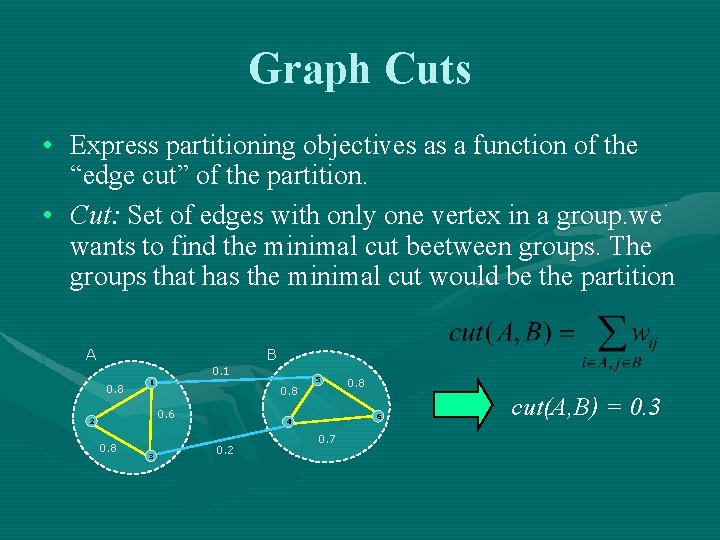Graph Cuts • Express partitioning objectives as a function of the “edge cut” of the partition. • Cut: Set of edges with only one vertex in a group. we wants to find the minimal cut beetween groups. The groups that has the minimal cut would be the partition A B 0. 1 0. 8 1 0. 6 2 0. 8 3 5 0. 8 6 4 0. 2 0. 8 0. 7 cut(A, B) = 0. 3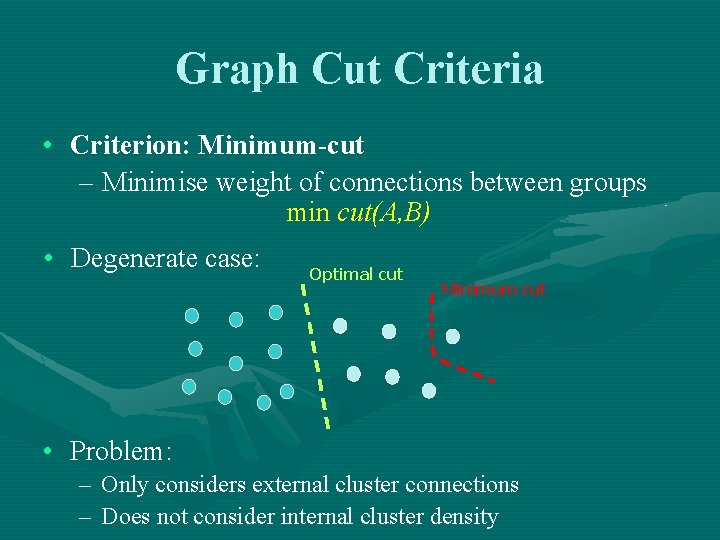Graph Cut Criteria • Criterion: Minimum-cut – Minimise weight of connections between groups min cut(A, B) • Degenerate case: Optimal cut Minimum cut • Problem: – Only considers external cluster connections – Does not consider internal cluster density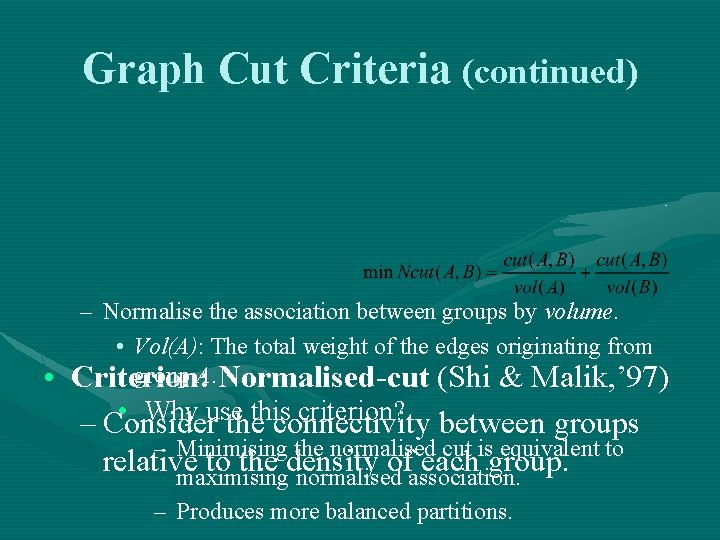Graph Cut Criteria (continued) • – Normalise the association between groups by volume. • Vol(A): The total weight of the edges originating from group A. Normalised-cut (Shi & Malik, ’ 97) Criterion: • Why use criterion? between groups – Consider thethis connectivity – Minimising the normalised cut is equivalent to relative to the density of each group. maximising normalised association. – Produces more balanced partitions.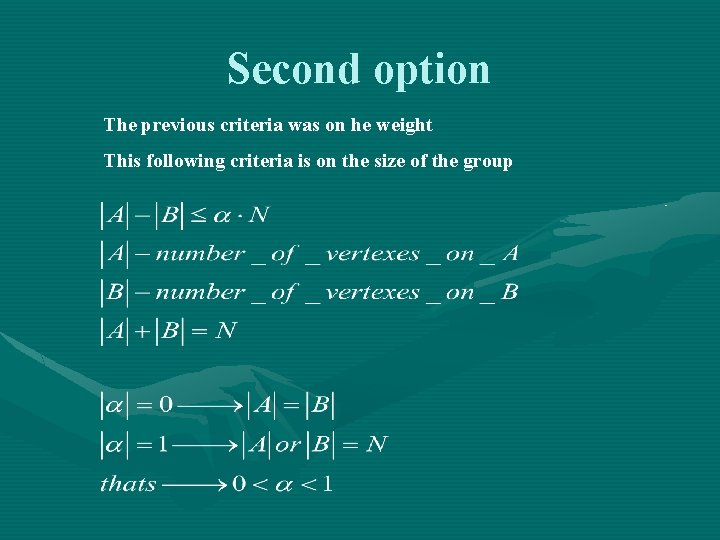Second option The previous criteria was on he weight This following criteria is on the size of the group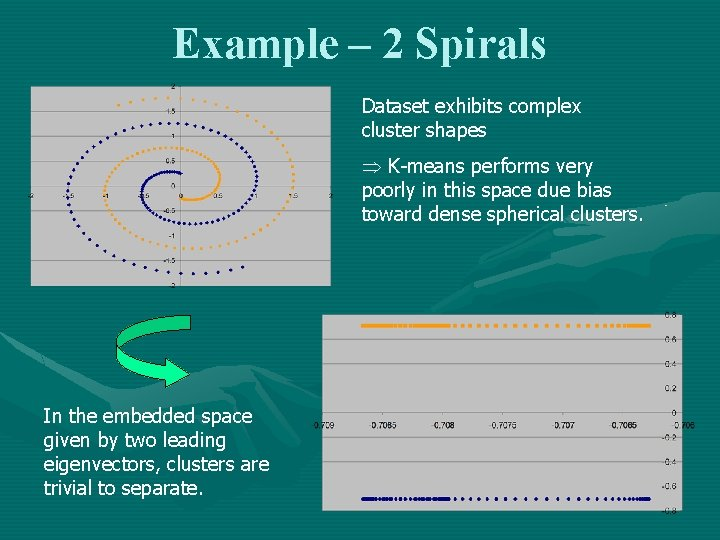Example – 2 Spirals Dataset exhibits complex cluster shapes Þ K-means performs very poorly in this space due bias toward dense spherical clusters. In the embedded space given by two leading eigenvectors, clusters are trivial to separate.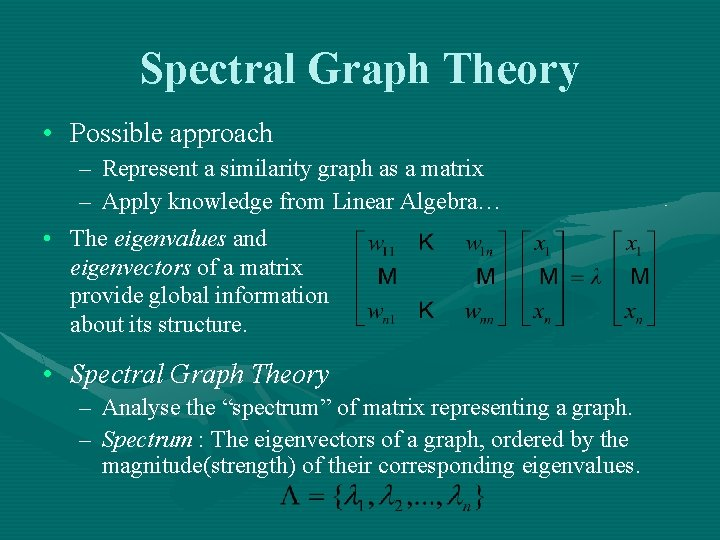Spectral Graph Theory • Possible approach – Represent a similarity graph as a matrix – Apply knowledge from Linear Algebra… • The eigenvalues and eigenvectors of a matrix provide global information about its structure. • Spectral Graph Theory – – Analyse the “spectrum” of matrix representing a graph. Spectrum : The eigenvectors of a graph, ordered by the magnitude(strength) of their corresponding eigenvalues.Matrix Representations • Adjacency matrix (A) – n x n matrix : edge weight between vertex xi and xj – 0. 1 0. 8 5 1 0. 8 0. 6 2 0. 8 6 4 0. 7 3 0. 2 x 1 x 2 x 3 x 4 x 5 x 6 x 1 0 0. 8 0. 6 0 0. 1 0 x 2 0. 8 0 0 0 x 3 0. 6 0. 8 0 0. 2 0 0 x 4 0 0 0. 2 0 0. 8 0. 7 x 5 0. 1 0 0 0. 8 x 6 0 0. 7 0. 8 0 • Important properties: – Symmetric matrix Þ Eigenvalues are real ÞEigenvector could span orthogonal base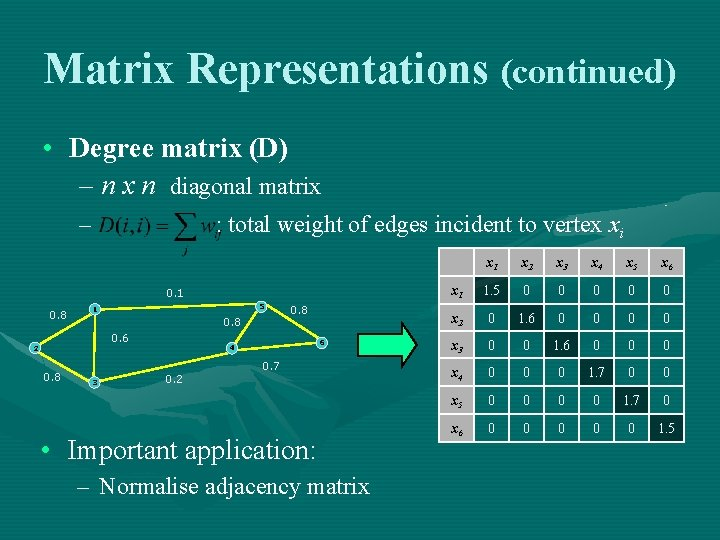Matrix Representations (continued) • Degree matrix (D) – n x n diagonal matrix – : total weight of edges incident to vertex xi 0. 1 0. 8 5 1 0. 8 0. 6 2 0. 8 3 0. 8 6 4 0. 2 0. 7 • Important application: – Normalise adjacency matrix x 1 x 2 x 3 x 4 x 5 x 6 x 1 1. 5 0 0 0 x 2 0 1. 6 0 0 x 3 0 0 1. 6 0 0 0 x 4 0 0 0 1. 7 0 0 x 5 0 0 1. 7 0 x 6 0 0 0 1. 5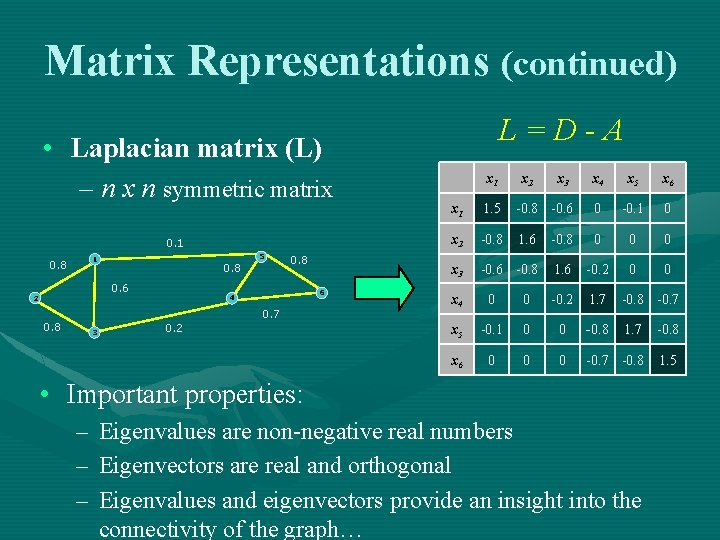Matrix Representations (continued) • Laplacian matrix (L) – n x n symmetric matrix 0. 1 5 1 0. 8 0. 6 2 0. 8 6 4 0. 7 0. 8 3 0. 2 L=D-A x 1 x 2 x 3 x 4 x 5 x 6 x 1 1. 5 -0. 8 -0. 6 0 -0. 1 0 x 2 -0. 8 1. 6 -0. 8 0 0 0 x 3 -0. 6 -0. 8 1. 6 -0. 2 0 0 x 4 0 0 -0. 2 1. 7 -0. 8 -0. 7 x 5 -0. 1 0 0 -0. 8 1. 7 -0. 8 x 6 0 0 0 -0. 7 -0. 8 1. 5 • Important properties: – – – Eigenvalues are non-negative real numbers Eigenvectors are real and orthogonal Eigenvalues and eigenvectors provide an insight into the connectivity of the graph…Another option – normalized laplasian 0. 1 0. 8 5 1 0. 8 0. 6 2 0. 8 • • 6 4 0. 7 3 0. 2 0. 8 1. 00 -0. 52 -0. 39 0. 00 -0. 06 0. 00 -0. 52 1. 00 -0. 50 0. 00 -0. 39 -0. 50 1. 00 -0. 12 0. 00 -0. 12 1. 00 -0. 47 -0. 44 -0. 06 0. 00 0. 47 - 1. 00 -0. 50 0. 00 -0. 44 -0. 50 1. 00 0. 00 Important properties: Laplacian matrix (L) – Eigenvectors are real and normalize n x n. Aij symmetric matrix –– Each (which i, j is not equal) =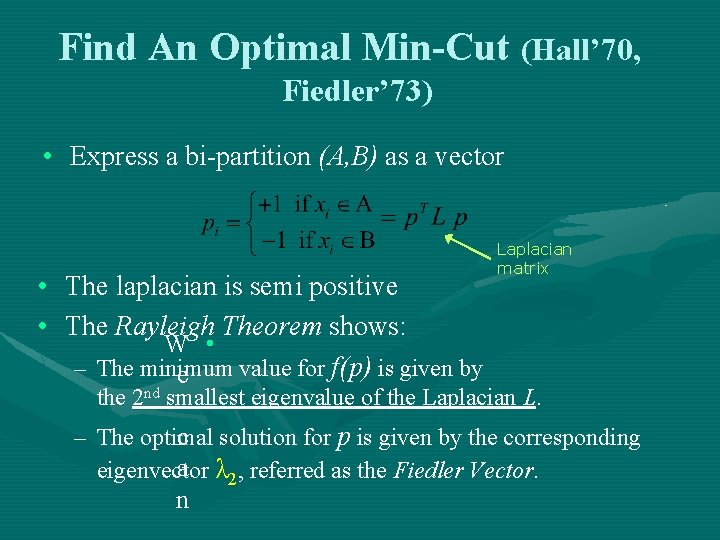Find An Optimal Min-Cut (Hall’ 70, Fiedler’ 73) • Express a bi-partition (A, B) as a vector • • The laplacian is semi positive The Rayleigh Theorem shows: W • – The minimum value for f(p) is given by e Laplacian matrix the 2 nd smallest eigenvalue of the Laplacian L. – The optimal c solution for p is given by the corresponding a λ 2, referred as the Fiedler Vector. eigenvector nProof • Based on • Consistency of Spectral Clustering By Ulrike von Luxburg 1, Mikhail Belkin 2, Olivier Bousquet Max Planck Institute for Biological Cybernetics Pages 2 -6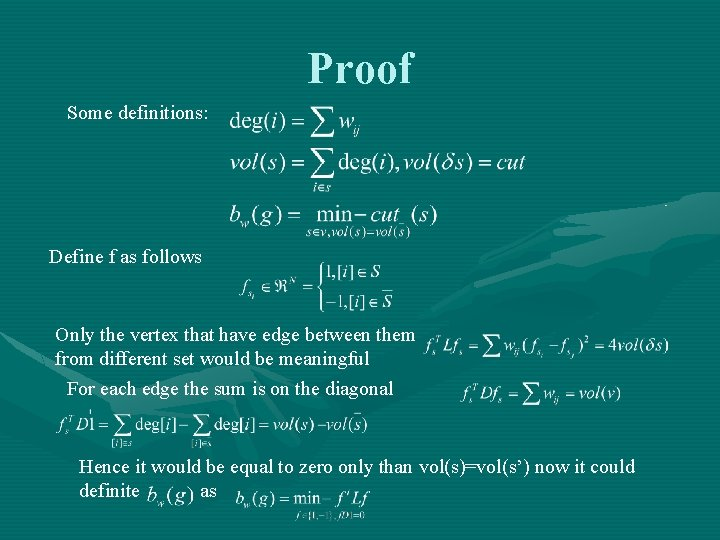Proof Some definitions: Define f as follows Only the vertex that have edge between them from different set would be meaningful For each edge the sum is on the diagonal Hence it would be equal to zero only than vol(s)=vol(s’) now it could definite asContinue … From simple algebra. . The relaxation method is for each vector on Eigen value worth Because the min of eigenvalue is 0 it doesn’t give us any information , and that’s why its bw(g)=2 nd eigenvalue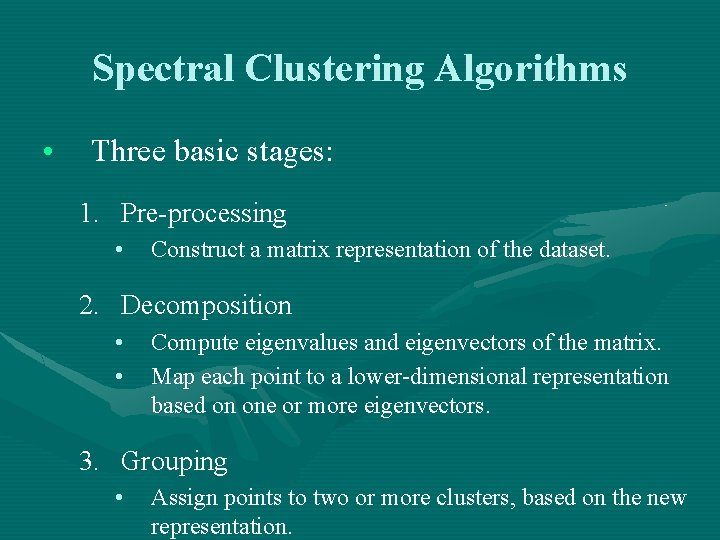Spectral Clustering Algorithms • Three basic stages: 1. Pre-processing • Construct a matrix representation of the dataset. 2. Decomposition • • Compute eigenvalues and eigenvectors of the matrix. Map each point to a lower-dimensional representation based on one or more eigenvectors. 3. Grouping • Assign points to two or more clusters, based on the new representation.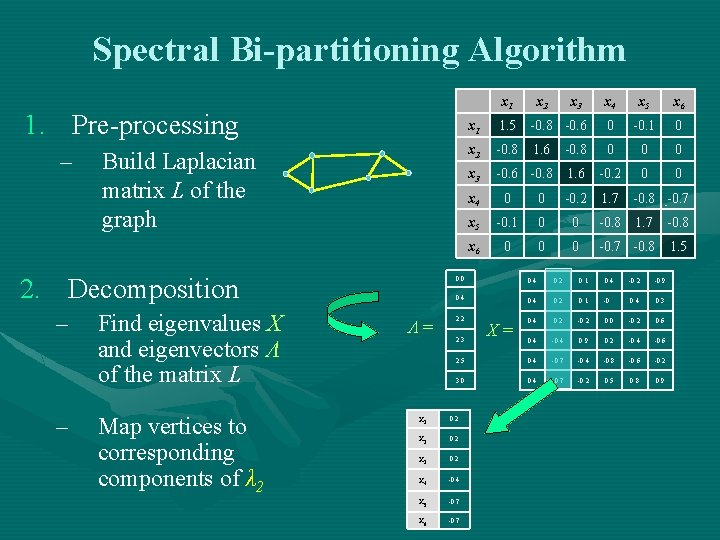Spectral Bi-partitioning Algorithm x 1 1. Pre-processing – Build Laplacian matrix L of the graph 2. Decomposition – – Find eigenvalues X and eigenvectors Λ of the matrix L Map vertices to corresponding components of λ 2 Λ= x 2 x 3 x 4 x 5 x 6 x 1 1. 5 -0. 8 -0. 6 0 -0. 1 0 x 2 -0. 8 1. 6 -0. 8 0 0 0 x 3 -0. 6 -0. 8 1. 6 -0. 2 0 0 x 4 0 0 -0. 2 1. 7 -0. 8 -0. 7 x 5 -0. 1 0 0 -0. 8 1. 7 -0. 8 x 6 0 0 0 -0. 7 -0. 8 1. 5 0. 0 0. 4 0. 2 0. 1 0. 4 -0. 2 -0. 9 0. 4 0. 2 0. 1 -0. 0. 4 0. 3 0. 4 0. 2 -0. 2 0. 0 -0. 2 0. 6 0. 4 -0. 4 0. 9 0. 2 -0. 4 -0. 6 2. 5 0. 4 -0. 7 -0. 4 -0. 8 -0. 6 -0. 2 3. 0 0. 4 -0. 7 -0. 2 0. 5 0. 8 0. 9 2. 2 2. 3 x 1 0. 2 x 2 0. 2 x 3 0. 2 x 4 -0. 4 x 5 -0. 7 x 6 -0. 7 X=Spectral Bi-partitioning Algorithm The matrix which represents the eigenvector of the laplacian the eigenvector matched to the corresponded eigenvalues with increasing order 0. 41 -0. 65 -0. 31 -0. 38 0. 11 0. 41 -0. 44 0. 01 0. 30 0. 71 0. 22 0. 41 -0. 37 0. 64 0. 04 -0. 39 -0. 37 0. 41 0. 37 0. 34 -0. 45 0. 00 0. 61 0. 41 -0. 17 -0. 30 0. 35 -0. 65 0. 41 0. 45 -0. 18 0. 72 -0. 29 0. 09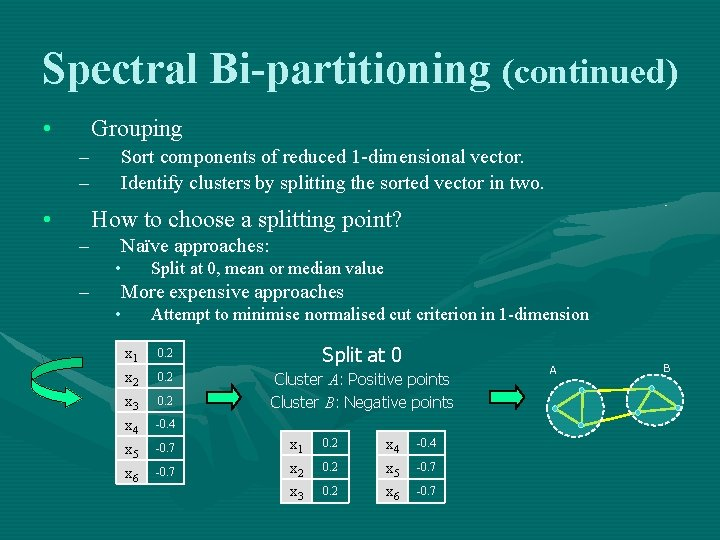Spectral Bi-partitioning (continued) • Grouping – – • Sort components of reduced 1 -dimensional vector. Identify clusters by splitting the sorted vector in two. How to choose a splitting point? – Naïve approaches: • – Split at 0, mean or median value More expensive approaches • Attempt to minimise normalised cut criterion in 1 -dimension x 1 x 2 x 3 x 4 x 5 x 6 0. 2 Split at 0 0. 2 Cluster A: Positive points Cluster B: Negative points 0. 2 -0. 4 -0. 7 x 1 x 2 x 3 0. 2 x 4 x 5 x 6 -0. 4 -0. 7 A B3 -Clusters Lets assume the next data points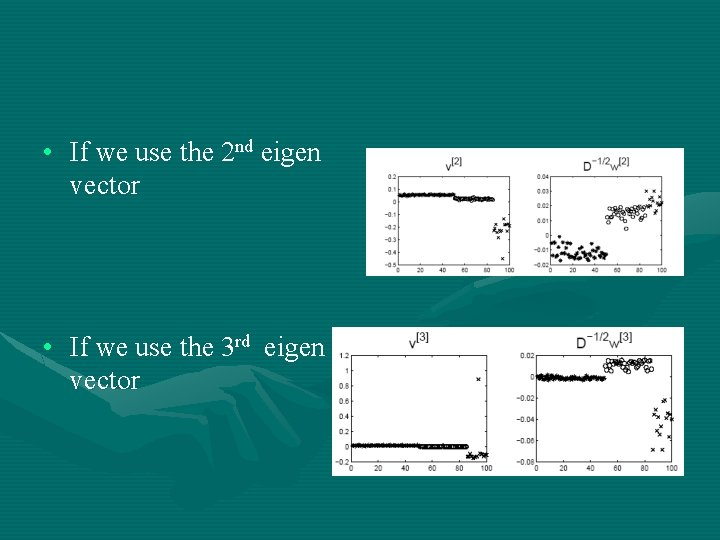• If we use the 2 nd eigen vector • If we use the 3 rd eigen vector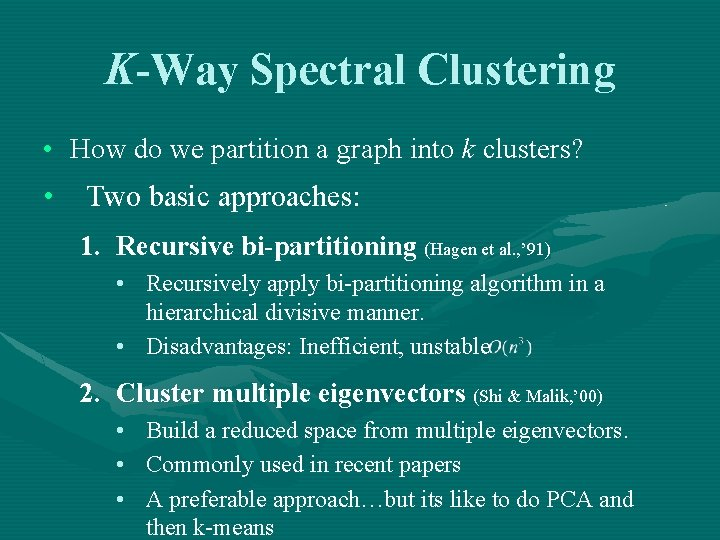K-Way Spectral Clustering • How do we partition a graph into k clusters? • Two basic approaches: 1. Recursive bi-partitioning (Hagen et al. , ’ 91) • Recursively apply bi-partitioning algorithm in a hierarchical divisive manner. • Disadvantages: Inefficient, unstable 2. Cluster multiple eigenvectors (Shi & Malik, ’ 00) • Build a reduced space from multiple eigenvectors. • Commonly used in recent papers • A preferable approach…but its like to do PCA and then k-meansRecursive bi-partitioning (Hagen et al. , ’ 91) • Partition using only one eigenvector at a time • Use procedure recursively • Example: Image Segmentation – Uses 2 nd (smallest) eigenvector to define optimal cut – Recursively generates two clusters with each cutWhy use Multiple Eigenvectors? 1. Approximates the optimal cut (Shi & Malik, ’ 00) – Can be used to approximate the optimal k-way normalised cut. 2. Emphasises cohesive clusters (Brand & Huang, ’ 02) – Increases the unevenness in the distribution of the data. Þ Associations between similar points are amplified, associations between dissimilar points are attenuated. Þ The data begins to “approximate a clustering”. 3. Well-separated space – Transforms data to a new “embedded space”, consisting of k orthogonal basis vectors. • NB: Multiple eigenvectors prevent instability due toK-Eigenvector Clustering • K-eigenvector Algorithm (Ng et al. , ’ 01) 1. Pre-processing – Construct the scaled adjacency matrix 2. Decomposition • • Find the eigenvalues and eigenvectors of A'. Build embedded space from the eigenvectors corresponding to the k largest eigenvalues. 3. Grouping • Apply k-means to reduced n x k space to produce k clusters.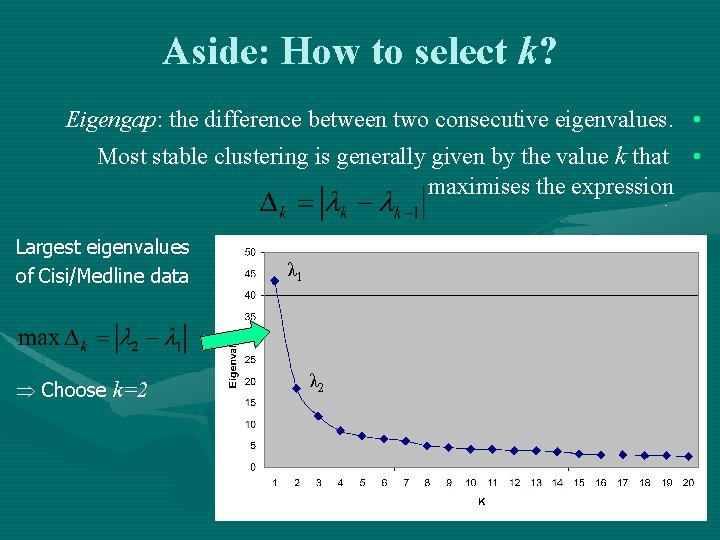Aside: How to select k? Eigengap: the difference between two consecutive eigenvalues. • Most stable clustering is generally given by the value k that • maximises the expression Largest eigenvalues of Cisi/Medline data Þ Choose k=2 λ 1 λ 2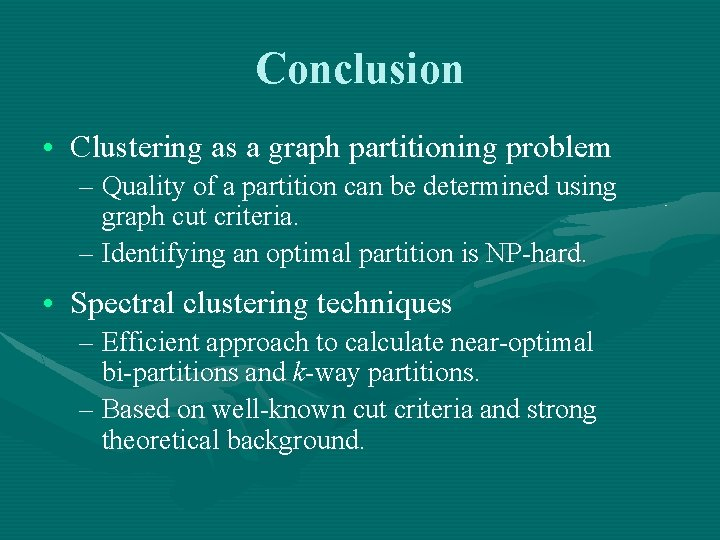Conclusion • Clustering as a graph partitioning problem – Quality of a partition can be determined using graph cut criteria. – Identifying an optimal partition is NP-hard. • Spectral clustering techniques – Efficient approach to calculate near-optimal bi-partitions and k-way partitions. – Based on well-known cut criteria and strong theoretical background.Selecting relevant genes with spectral approach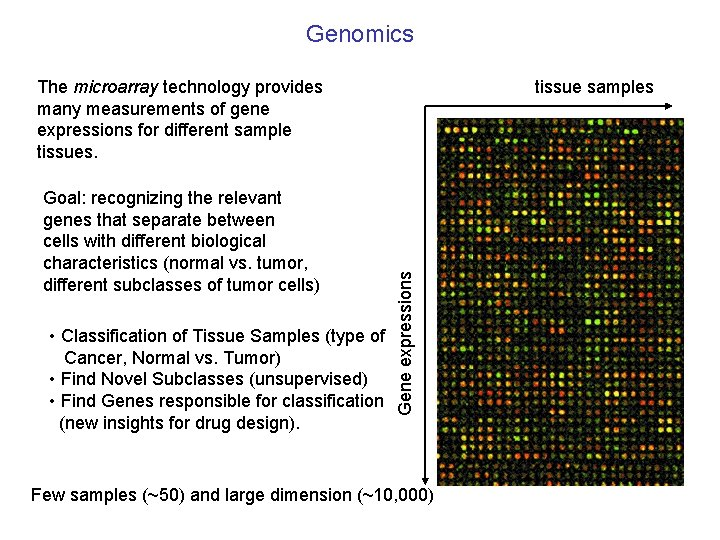Genomics Goal: recognizing the relevant genes that separate between cells with different biological characteristics (normal vs. tumor, different subclasses of tumor cells) • Classification of Tissue Samples (type of Cancer, Normal vs. Tumor) • Find Novel Subclasses (unsupervised) • Find Genes responsible for classification (new insights for drug design). tissue samples Gene expressions The microarray technology provides many measurements of gene expressions for different sample tissues. Few samples (~50) and large dimension (~10, 000)Problem Definition lets the microarray data matrix define by M The gene expression levels form the rows of M Normalized gene vector which are most “relevant” with respect to an inference (learning) task. tissue vector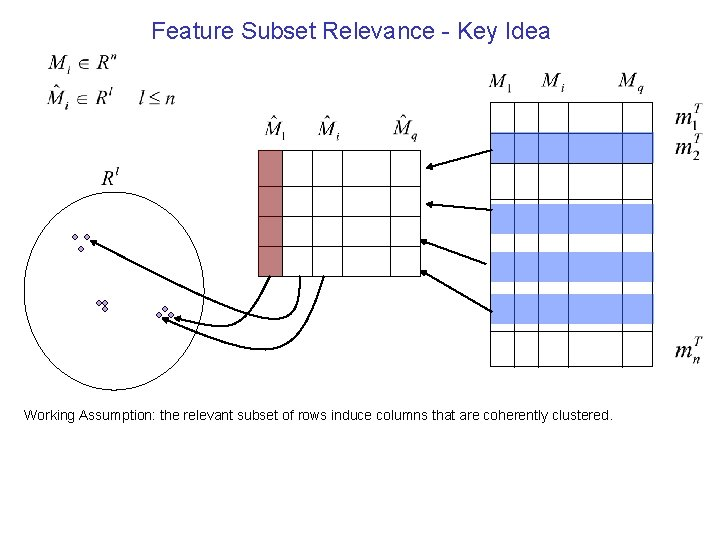Feature Subset Relevance - Key Idea Working Assumption: the relevant subset of rows induce columns that are coherently clustered.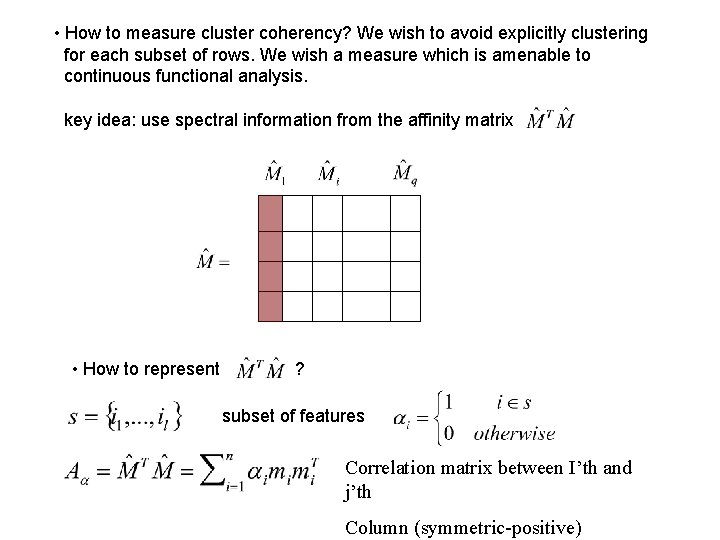• How to measure cluster coherency? We wish to avoid explicitly clustering for each subset of rows. We wish a measure which is amenable to continuous functional analysis. key idea: use spectral information from the affinity matrix • How to represent ? subset of features Correlation matrix between I’th and j’th Column (symmetric-positive)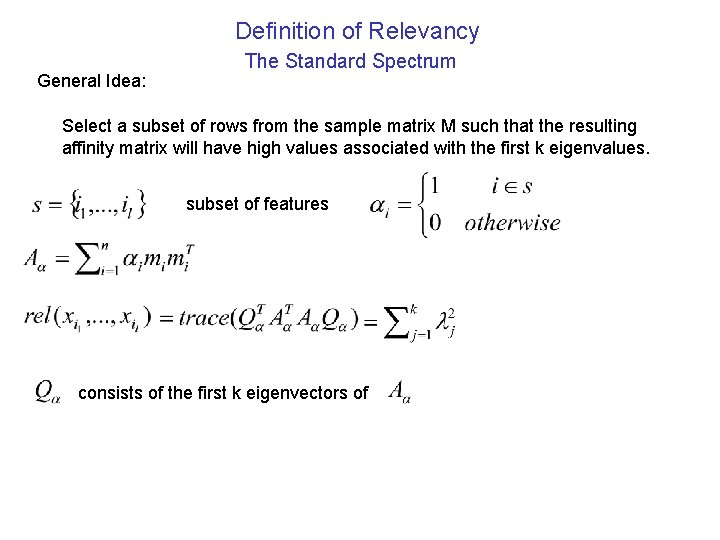Definition of Relevancy General Idea: The Standard Spectrum Select a subset of rows from the sample matrix M such that the resulting affinity matrix will have high values associated with the first k eigenvalues. subset of features consists of the first k eigenvectors of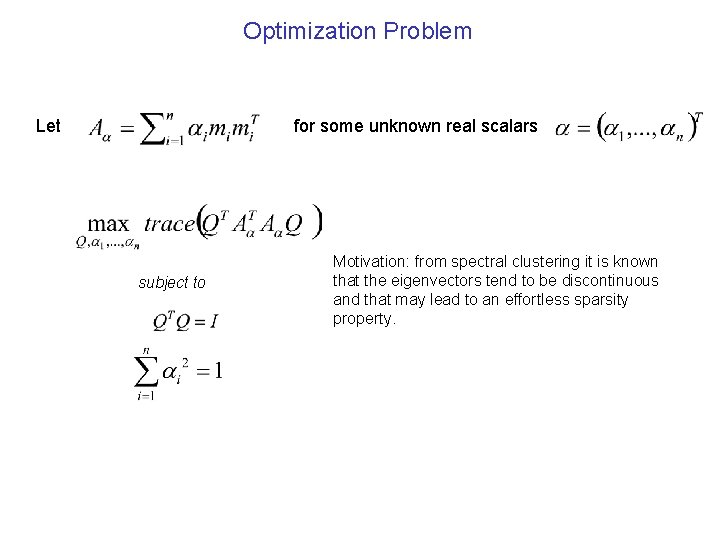Optimization Problem Let for some unknown real scalars subject to Motivation: from spectral clustering it is known that the eigenvectors tend to be discontinuous and that may lead to an effortless sparsity property.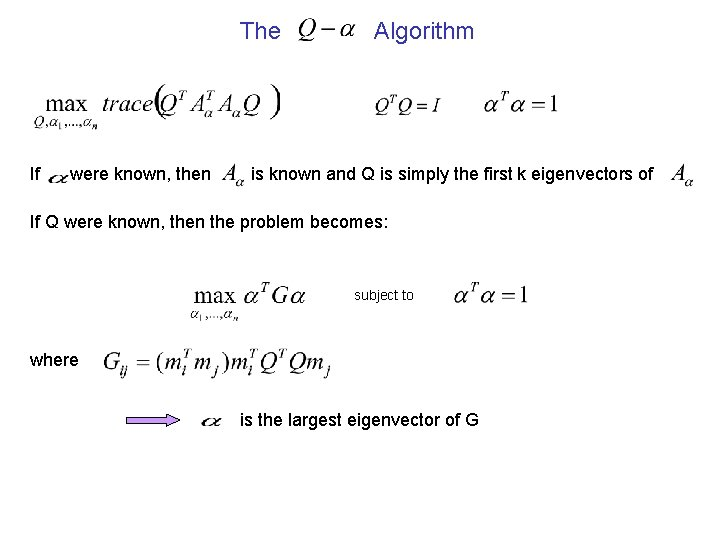The If were known, then Algorithm is known and Q is simply the first k eigenvectors of If Q were known, then the problem becomes: subject to where is the largest eigenvector of G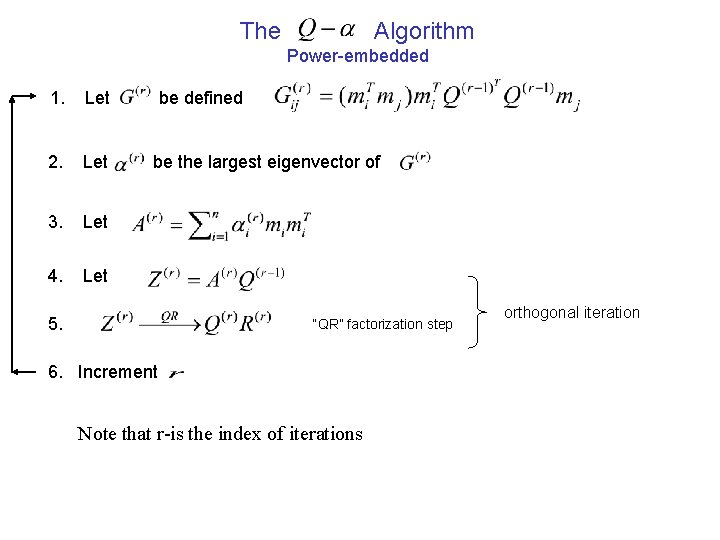The Algorithm Power-embedded 1. Let 2. Let 3. Let 4. Let be defined be the largest eigenvector of 5. “QR” factorization step 6. Increment Note that r-is the index of iterations orthogonal iterationThe algorithm need 3 conditions: • the algorithm converges to a local maximum • At the local maximum • The vector is sparseThe experiment • Giving some data sets: blood cell • Myeloid cell: HL-60 U 937. • T cell-Jurkat, • Leukemia cell NB 4 • The dimensionality of the expression data was 7229 genes over 17 sampeles • The goal is to find cluster of the expression level of the gene without any restriction.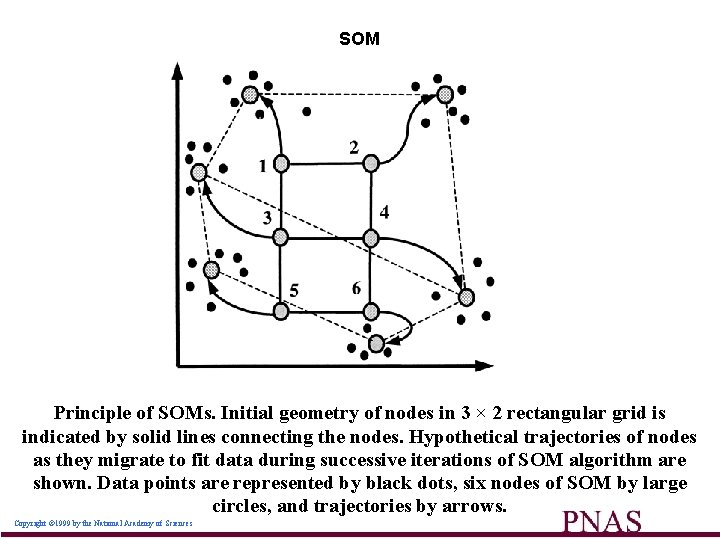SOM Principle of SOMs. Initial geometry of nodes in 3 × 2 rectangular grid is indicated by solid lines connecting the nodes. Hypothetical trajectories of nodes as they migrate to fit data during successive iterations of SOM algorithm are shown. Data points are represented by black dots, six nodes of SOM by large circles, and trajectories by arrows. Copyright © 1999 by the National Academy of Sciences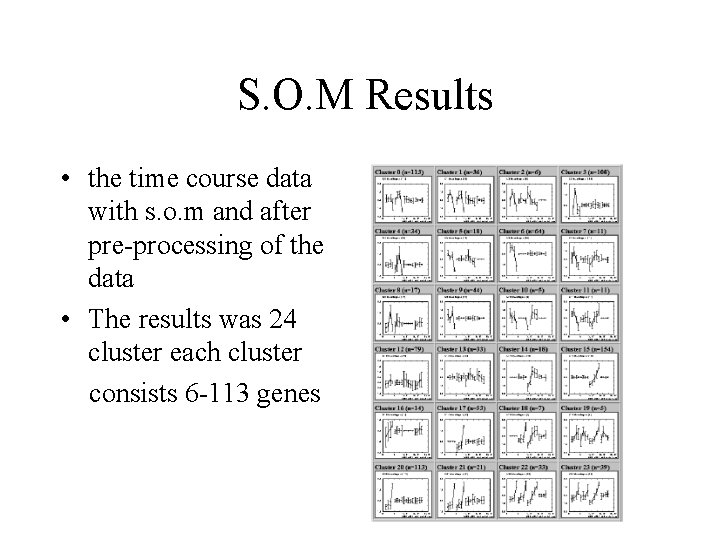S. O. M Results • the time course data with s. o. m and after pre-processing of the data • The results was 24 cluster each cluster consists 6 -113 genes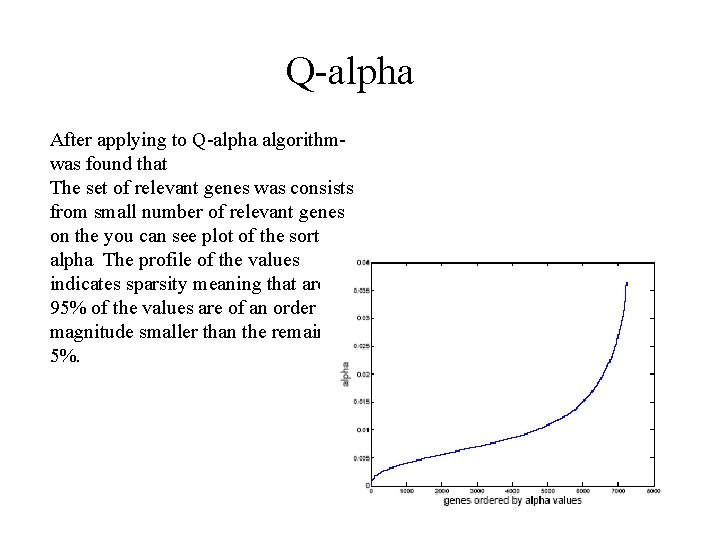Q-alpha After applying to Q-alpha algorithmwas found that The set of relevant genes was consists from small number of relevant genes on the you can see plot of the sorted – alpha The profile of the values indicates sparsity meaning that around 95% of the values are of an order of magnitude smaller than the remaining 5%.continue A plot of 6 of the top 40 genes that correspond to clusters 20, 1, 22/23, 4, 15, 21 In each of the six panels time courses of all four cell lines are shown (left to right) HL-60, U 937, NB 4, Jurkat.Another example • Another dataset : 1. DLCL, reffered to as ”lymphoma” 7, 129 genes over 56 samples 2. Childhood medulloblastomas referred to as ”brain”. The dimensionality of this dataset was 7, 129 and there were 60 samples 3. Breast tumors reffered to as ”breast met”. The dimensionality of this dataset was 24, 624 and there were 44 samples where 4. The fourth breast tumors for which corresponding lymph nodes either were cancerous or not, referred to as ”lymph status”. The dimensionality of this dataset is 12, 600 with 90 samples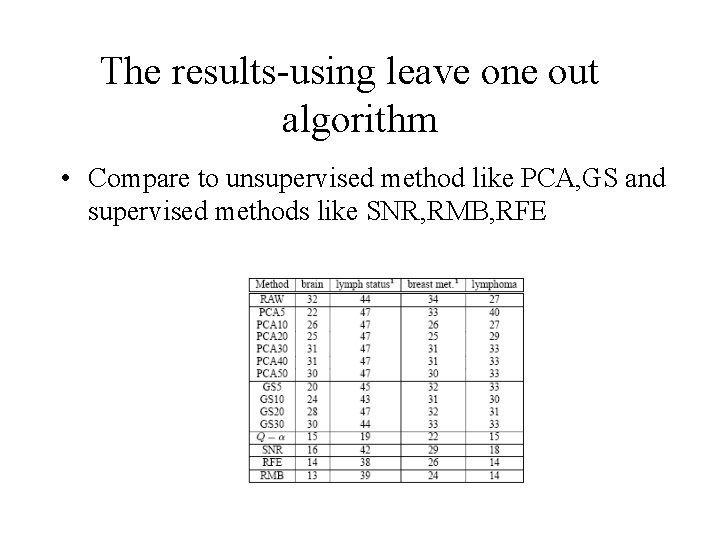The results-using leave one out algorithm • Compare to unsupervised method like PCA, GS and supervised methods like SNR, RMB, RFEThe slide you all waited for